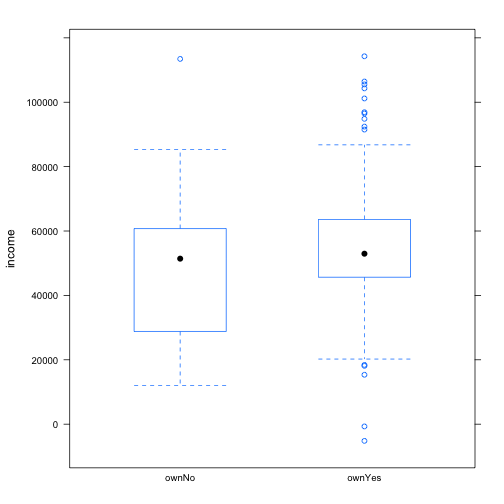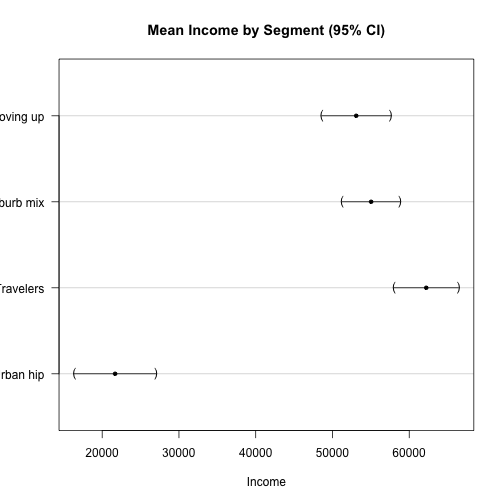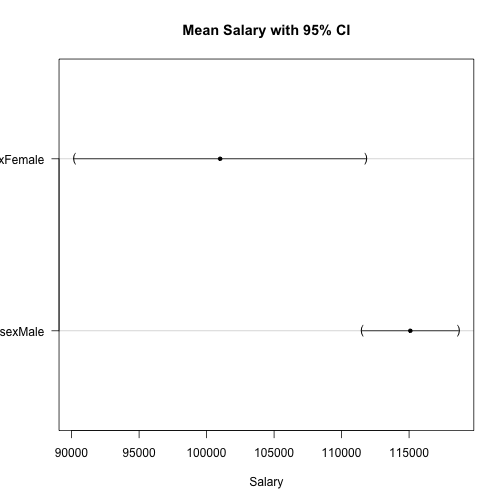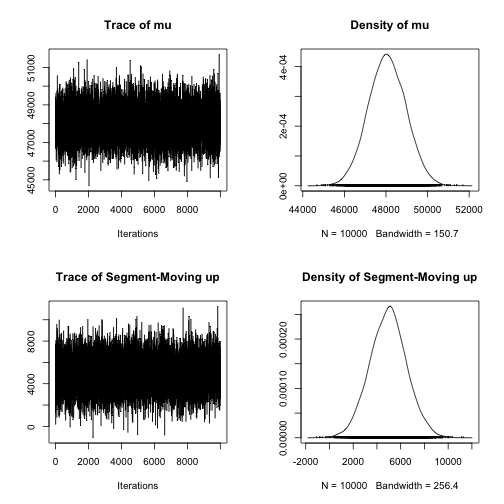# R for Marketing Research and Analytics

Chris Chapman and Elea McDonnell Feit
January 2016

Chapter 6: Statistics to Compare Groups

Website for all data files:
http://r-marketing.r-forge.r-project.org/data.html

### Load the data (same as Chapter 5)

As always, see the book for details on the data simulation:

``````seg.df <- read.csv("http://goo.gl/qw303p")
summary(seg.df)
``````
``````      age           gender        income            kids        ownHome
Min.   :19.26   Female:157   Min.   : -5183   Min.   :0.00   ownNo :159
1st Qu.:33.01   Male  :143   1st Qu.: 39656   1st Qu.:0.00   ownYes:141
Median :39.49                Median : 52014   Median :1.00
Mean   :41.20                Mean   : 50937   Mean   :1.27
3rd Qu.:47.90                3rd Qu.: 61403   3rd Qu.:2.00
Max.   :80.49                Max.   :114278   Max.   :7.00
subscribe         Segment
subNo :260   Moving up : 70
subYes: 40   Suburb mix:100
Travelers : 80
Urban hip : 50

``````

### Chi-square test

Tests equality of marginal counts in groups. Important: compile a table first (don't use raw data). Then use `chisq.test()`.

Let's see this for simple, fake data first:

``````tmp.tab <- table(rep(c(1:4), times=c(25,25,25,20)))
tmp.tab
``````
``````
1  2  3  4
25 25 25 20
``````
``````chisq.test(tmp.tab)
``````
``````
Chi-squared test for given probabilities

data:  tmp.tab
X-squared = 0.78947, df = 3, p-value = 0.852
``````

### chisq.test "significant" and "not significant"

``````tmp.tab <- table(rep(c(1:4), times=c(25,25,25,20)))
chisq.test(tmp.tab)
``````
``````
Chi-squared test for given probabilities

data:  tmp.tab
X-squared = 0.78947, df = 3, p-value = 0.852
``````
``````tmp.tab <- table(rep(c(1:4), times=c(25,25,25,10)))
tmp.tab
``````
``````
1  2  3  4
25 25 25 10
``````
``````chisq.test(tmp.tab)
``````
``````
Chi-squared test for given probabilities

data:  tmp.tab
X-squared = 7.9412, df = 3, p-value = 0.04724
``````

### chisq.test with segment data

Are the segments the same size? (Not a very interesting question, perhaps.)

``````table(seg.df\$Segment)
``````
``````
Moving up Suburb mix  Travelers  Urban hip
70        100         80         50
``````
``````chisq.test(table(seg.df\$Segment))
``````
``````
Chi-squared test for given probabilities

data:  table(seg.df\$Segment)
X-squared = 17.333, df = 3, p-value = 0.0006035
``````

### chisq.test with segment data

Do they have the same rate of subscription by ownership?

``````table(seg.df\$subscribe, seg.df\$ownHome)
``````
``````
ownNo ownYes
subNo    137    123
subYes    22     18
``````
``````chisq.test(table(seg.df\$subscribe, seg.df\$ownHome))
``````
``````
Pearson's Chi-squared test with Yates' continuity correction

data:  table(seg.df\$subscribe, seg.df\$ownHome)
X-squared = 0.010422, df = 1, p-value = 0.9187
``````

``````chisq.test(table(seg.df\$subscribe, seg.df\$ownHome), correct=FALSE)
``````
``````
Pearson's Chi-squared test

data:  table(seg.df\$subscribe, seg.df\$ownHome)
X-squared = 0.074113, df = 1, p-value = 0.7854
``````

### Proportions: binomial test

`binom.test(x=successes, n=trials, p=proportion)` tests whether the count of successes in a certain number of trials matches an expected proportion:

``````binom.test(12, 20, p=0.5)
``````
``````
Exact binomial test

data:  12 and 20
number of successes = 12, number of trials = 20, p-value = 0.5034
alternative hypothesis: true probability of success is not equal to 0.5
95 percent confidence interval:
0.3605426 0.8088099
sample estimates:
probability of success
0.6
``````

### Proportions: binomial test continued

The same proportion with higher N can be significant:

``````# binom.test(12, 20, p=0.5)
binom.test(120, 200, p=0.5)
``````
``````
Exact binomial test

data:  120 and 200
number of successes = 120, number of trials = 200, p-value =
0.005685
alternative hypothesis: true probability of success is not equal to 0.5
95 percent confidence interval:
0.5285357 0.6684537
sample estimates:
probability of success
0.6
``````

See book for Agresti-Coull and other methods if your data has small N or is near 0 or 1 proportion.

### t-tests

Does income differ for home owners in our data? A t-test compares the means of two groups, relative to the variance. First let's visualize it:

``````library(lattice)
bwplot(income ~ ownHome, data=seg.df)
``````### t.test()

Use formula syntax `t.test(outcomeVar ~ groupingVar)`:

``````t.test(income ~ ownHome, data=seg.df)
``````
``````
Welch Two Sample t-test

data:  income by ownHome
t = -3.2731, df = 285.25, p-value = 0.001195
alternative hypothesis: true difference in means is not equal to 0
95 percent confidence interval:
-12080.155  -3007.193
sample estimates:
mean in group ownNo mean in group ownYes
47391.01             54934.68
``````

Mean income is higher among home owners in our data, p < .01.

### t.test() for a subset() of data

`subset()` is an easy way to select portions of a data set. Here's the same t-test but only for the Travelers segment:

``````t.test(income ~ ownHome, data=subset(seg.df, Segment=="Travelers"))
``````
``````
Welch Two Sample t-test

data:  income by ownHome
t = 0.26561, df = 53.833, p-value = 0.7916
alternative hypothesis: true difference in means is not equal to 0
95 percent confidence interval:
-8508.993 11107.604
sample estimates:
mean in group ownNo mean in group ownYes
63188.42             61889.12
``````

Mean income is not significantly different between home owners and non-owners in the Travelers segment.

### ANOVA basics

ANOVA (analysis of variance) compares the difference in means among two or more groups, relative to their variances.

Recommended procedure: (1) fit a model with `aov()`*. (2) use *`anova()` on the model object to obtain a typical ANOVA table.

For two groups it is effectively the same as a t-test:

``````seg.aov.own <- aov(income ~ ownHome, data=seg.df)
anova(seg.aov.own)
``````
``````Analysis of Variance Table

Response: income
Df     Sum Sq    Mean Sq F value   Pr(>F)
ownHome     1 4.2527e+09 4252661211  10.832 0.001118 **
Residuals 298 1.1700e+11  392611030
---
Signif. codes:  0 '***' 0.001 '**' 0.01 '*' 0.05 '.' 0.1 ' ' 1
``````

### ANOVA: Multiple groups

The same model works for multiple groups. Make sure that the independent variable is a factor (use `factor()` to convert if necessary).

``````aggregate(income ~ Segment, mean, data=seg.df)
``````
``````     Segment   income
1  Moving up 53090.97
2 Suburb mix 55033.82
3  Travelers 62213.94
4  Urban hip 21681.93
``````
``````seg.aov.seg <- aov(income ~ Segment, data=seg.df)
anova(seg.aov.seg)
``````
``````Analysis of Variance Table

Response: income
Df     Sum Sq    Mean Sq F value    Pr(>F)
Segment     3 5.4970e+10 1.8323e+10  81.828 < 2.2e-16 ***
Residuals 296 6.6281e+10 2.2392e+08
---
Signif. codes:  0 '***' 0.001 '**' 0.01 '*' 0.05 '.' 0.1 ' ' 1
``````

### Multiple Effect Models: Basic Formulas

Use formula syntax to add variables

Symbol Meaning
~ RESPONSE ~ PREDICTOR(s)
: Interaction without main effect
* All main effects and their interactions
. Shortcut for “all other variables”

Examples:

Model Formula
income by ownership & segment income ~ own + segment
income by interaaction of ownership by segment income ~ own:segment
income with all effects of ownership with segment income ~ own + segment + own:segment
… or the same but not as clear … income ~ own*segment
income as a response to all other variables income ~ .

### ANOVA with Segment + Ownership

``````anova(aov(income ~ Segment + ownHome, data=seg.df))
``````
``````Analysis of Variance Table

Response: income
Df     Sum Sq    Mean Sq F value Pr(>F)
Segment     3 5.4970e+10 1.8323e+10 81.6381 <2e-16 ***
ownHome     1 6.9918e+07 6.9918e+07  0.3115 0.5772
Residuals 295 6.6211e+10 2.2444e+08
---
Signif. codes:  0 '***' 0.001 '**' 0.01 '*' 0.05 '.' 0.1 ' ' 1
``````

Mean income differs by Segment, but not by ownership after Segment is controlled. Model by ownership alone might be misleading:

``````anova(aov(income ~ ownHome, data=seg.df))
``````
``````Analysis of Variance Table

Response: income
Df     Sum Sq    Mean Sq F value   Pr(>F)
ownHome     1 4.2527e+09 4252661211  10.832 0.001118 **
Residuals 298 1.1700e+11  392611030
---
Signif. codes:  0 '***' 0.001 '**' 0.01 '*' 0.05 '.' 0.1 ' ' 1
``````

### ANOVA with interaction

``````anova(aov(income ~ Segment * ownHome, data=seg.df))
``````
``````Analysis of Variance Table

Response: income
Df     Sum Sq    Mean Sq F value Pr(>F)
Segment           3 5.4970e+10 1.8323e+10 81.1305 <2e-16 ***
ownHome           1 6.9918e+07 6.9918e+07  0.3096 0.5784
Segment:ownHome   3 2.6329e+08 8.7762e+07  0.3886 0.7613
Residuals       292 6.5948e+10 2.2585e+08
---
Signif. codes:  0 '***' 0.001 '**' 0.01 '*' 0.05 '.' 0.1 ' ' 1
``````

Mean income differs by segment, not by ownership, and not by the interaction of ownership with segment.

Recommended: instead of using * , specify all effects directly:

``````anova(aov(income ~ Segment + ownHome + Segment:ownHome, data=seg.df))
``````

### Model Comparison

The `anova()` command will also compare the fit of models:

``````anova(aov(income ~ Segment,           data=seg.df),
aov(income ~ Segment + ownHome, data=seg.df))
``````
``````Analysis of Variance Table

Model 1: income ~ Segment
Model 2: income ~ Segment + ownHome
Res.Df        RSS Df Sum of Sq      F Pr(>F)
1    296 6.6281e+10
2    295 6.6211e+10  1  69918004 0.3115 0.5772
``````

In this case, once we model income by segment, adding ownership does not improve the model fit.

### Visualization: ANOVA Group means

Use glht() in multcomp package as an easy way to get mean and CI:

``````# install.packages("multcomp")     # if needed
library(multcomp)
seg.aov <- aov(income ~ -1 + Segment, data=seg.df)   # model w/o int.
by.seg  <- glht(seg.aov)                             # means and CIs
plot(by.seg, xlab="Income", main="Mean Income by Segment (95% CI)")
``````### Exercises (Basic)

Access the `Salaries` data set:

``````library(car)    # install.packages("car") if needed
data(Salaries)
``````
1. Does the proportion of women differ by discipline?
2. In a one-way ANOVA, are salaries different for men and women?
3. Visualize the mean salary for men and women, with 95% confidence intervals.

Does the proportion of women differ by discipline?

``````with(Salaries, prop.table(table(discipline, sex), margin=1))
``````
``````          sex
discipline     Female       Male
A 0.09944751 0.90055249
B 0.09722222 0.90277778
``````
``````with(Salaries, chisq.test(table(discipline, sex)))
``````
``````
Pearson's Chi-squared test with Yates' continuity correction

data:  table(discipline, sex)
X-squared = 2.0875e-29, df = 1, p-value = 1
``````

In a one-way ANOVA, are salaries different for men and women?

``````aggregate(salary ~ sex, data=Salaries, mean)
``````
``````     sex   salary
1 Female 101002.4
2   Male 115090.4
``````
``````anova(aov(salary ~ sex, data=Salaries))
``````
``````Analysis of Variance Table

Response: salary
Df     Sum Sq    Mean Sq F value   Pr(>F)
sex         1 6.9800e+09 6980014930  7.7377 0.005667 **
Residuals 395 3.5632e+11  902077538
---
Signif. codes:  0 '***' 0.001 '**' 0.01 '*' 0.05 '.' 0.1 ' ' 1
``````

Visualize the mean salary for men and women, with 95% confidence intervals.

``````# install.packages("multcomp")     # if needed
library(multcomp)
salary.aov <- aov(salary ~ -1 + sex, data=Salaries)
by.sex  <- glht(salary.aov)
plot(by.sex, xlab="Salary", main="Mean Salary with 95% CI")
``````## Extra Slides

• Stepwise ANOVA
• Bayesian ANOVA

### Optional: Stepwise ANOVA

Use step() to do forward or (default) backward stepwise model fit. This fits models and successively drops (or adds) variables to see if fit improves. Returing to the segmentation data:

``````seg.aov.step <- step(aov(income ~ ., data=seg.df))
``````
``````Start:  AIC=5779.17
income ~ age + gender + kids + ownHome + subscribe + Segment

Df  Sum of Sq        RSS    AIC
- age        1 4.7669e+06 6.5661e+10 5777.2
- ownHome    1 1.0337e+08 6.5759e+10 5777.6
- kids       1 1.3408e+08 6.5790e+10 5777.8
- subscribe  1 1.5970e+08 6.5816e+10 5777.9
- gender     1 2.6894e+08 6.5925e+10 5778.4
<none>                    6.5656e+10 5779.2
- Segment    3 1.9303e+10 8.4959e+10 5850.5

Step:  AIC=5777.19
income ~ gender + kids + ownHome + subscribe + Segment

Df  Sum of Sq        RSS    AIC
- ownHome    1 1.0159e+08 6.5762e+10 5775.7
- kids       1 1.3205e+08 6.5793e+10 5775.8
- subscribe  1 1.5794e+08 6.5819e+10 5775.9
- gender     1 2.7009e+08 6.5931e+10 5776.4
<none>                    6.5661e+10 5777.2
- Segment    3 4.9044e+10 1.1470e+11 5938.6

Step:  AIC=5775.66
income ~ gender + kids + subscribe + Segment

Df  Sum of Sq        RSS    AIC
- kids       1 1.0707e+08 6.5869e+10 5774.1
- subscribe  1 1.6370e+08 6.5926e+10 5774.4
- gender     1 2.5520e+08 6.6017e+10 5774.8
<none>                    6.5762e+10 5775.7
- Segment    3 5.2897e+10 1.1866e+11 5946.7

Step:  AIC=5774.15
income ~ gender + subscribe + Segment

Df  Sum of Sq        RSS    AIC
- subscribe  1 1.6226e+08 6.6032e+10 5772.9
- gender     1 2.4390e+08 6.6113e+10 5773.3
<none>                    6.5869e+10 5774.1
- Segment    3 5.3005e+10 1.1887e+11 5945.3

Step:  AIC=5772.88
income ~ gender + Segment

Df  Sum of Sq        RSS    AIC
- gender   1 2.4949e+08 6.6281e+10 5772.0
<none>                  6.6032e+10 5772.9
- Segment  3 5.4001e+10 1.2003e+11 5946.2

Step:  AIC=5772.02
income ~ Segment

Df Sum of Sq        RSS    AIC
<none>                 6.6281e+10 5772.0
- Segment  3 5.497e+10 1.2125e+11 5947.2
``````

### Stepwise ANOVA: Result

``````seg.aov.step <- step(aov(income ~ ., data=seg.df))
``````

After step() tests all main effect variables in the model (income ~ .), the best-fitting model balancing fit and complexity is:

``````anova(seg.aov.step)
``````
``````Analysis of Variance Table

Response: income
Df     Sum Sq    Mean Sq F value    Pr(>F)
Segment     3 5.4970e+10 1.8323e+10  81.828 < 2.2e-16 ***
Residuals 296 6.6281e+10 2.2392e+08
---
Signif. codes:  0 '***' 0.001 '**' 0.01 '*' 0.05 '.' 0.1 ' ' 1
``````

There are many R packages that support Bayesian inference. Simple example for ANOVA. Fit two models:

``````# install.packages("BayesFactor")   # if needed
library(BayesFactor)
set.seed(96761)                     # optional, for replication
seg.bf1 <- lmBF(income ~ Segment,           data=seg.df)
seg.bf2 <- lmBF(income ~ Segment + ownHome, data=seg.df)
``````

And compare them for which has more evidence in the data:

``````seg.bf1 / seg.bf2
``````
``````Bayes factor analysis
--------------
 Segment : 6.579729 ±1.62%

Against denominator:
income ~ Segment + ownHome
---
Bayes factor type: BFlinearModel, JZS
``````

Model 1 has “6x” as much evidence, considering just these two models.

### Bayesian ANOVA: Under the hood

The model works by drawing 1000s of likely estimates for the model parameters (overall mean and segment means). We can examine:

``````seg.bf.chain <- posterior(seg.bf1, 1, iterations = 10000)
``````
``````Markov Chain Monte Carlo (MCMC) output:
Start = 1
End = 7
Thinning interval = 1
mu Segment-Moving up Segment-Suburb mix Segment-Travelers
[1,] 48055.75         4964.3105           6909.032          13983.21
[2,] 47706.52         6478.1497           7816.873          12160.32
[3,] 48362.90         5228.0718           6654.030          12565.87
[4,] 49417.43         5300.9543           7249.228          12218.89
[5,] 48177.21         6150.6339           6025.763          15589.03
[6,] 49440.51          186.6233           6259.676          14172.76
[7,] 46650.93         3530.4188           6921.267          14348.78
``````

Segment estimates are deviations from the overall mean estimate.

### Bayesian ANOVA: Plotting the Draws

``````plot(seg.bf.chain[, 1:2])   # overall mean + first segment
``````### Bayesian ANOVA: Segment Estimates

Segment mean estimate = overall mean + segment deviation

``````seg.bf.chain[1:4, 1:4]
``````
``````           mu Segment-Moving up Segment-Suburb mix Segment-Travelers
[1,] 48055.75          4964.310           6909.032          13983.21
[2,] 47706.52          6478.150           7816.873          12160.32
[3,] 48362.90          5228.072           6654.030          12565.87
[4,] 49417.43          5300.954           7249.228          12218.89
``````
``````seg.bf.chain[1:4, 2:4] + seg.bf.chain[1:4, 1]
``````
``````     Segment-Moving up Segment-Suburb mix Segment-Travelers
[1,]          53020.06           54964.78          62038.95
[2,]          54184.67           55523.40          59866.84
[3,]          53590.97           55016.93          60928.77
[4,]          54718.38           56666.66          61636.32
``````

### Bayesian ANOVA: Segment CIs

First get the segment estimates for each of 10000 draws:

``````seg.bf.chain.total <- seg.bf.chain[, 2:5] + seg.bf.chain[, 1]
seg.bf.chain.total[1:4, 1:3]
``````
``````     Segment-Moving up Segment-Suburb mix Segment-Travelers
[1,]          53020.06           54964.78          62038.95
[2,]          54184.67           55523.40          59866.84
[3,]          53590.97           55016.93          60928.77
[4,]          54718.38           56666.66          61636.32
``````

Then get the 95% credible intervals that we observe there:

``````seg.bf.ci <- t(apply(seg.bf.chain.total, 2,
quantile, pr=c(0.025, 0.5, 0.975)))
seg.bf.ci
``````
``````                       2.5%      50%    97.5%
Segment-Moving up  49582.08 53020.98 56522.05
Segment-Suburb mix 52039.66 54988.99 57867.29
Segment-Travelers  58799.46 62048.33 65355.62
Segment-Urban hip  17992.85 22216.26 26450.56
``````

### Bayesian ANOVA: Plot the CIs

Make a data frame of the CI results:

``````seg.bf.df <- data.frame(seg.bf.ci)
seg.bf.df\$Segment <- rownames(seg.bf.df)
``````

And use that to build the plot:

``````library(ggplot2)
# basic plot object with CIs on Y axis by Segment on X
p <- ggplot(seg.bf.df, aes(x=Segment,
y=X50., ymax=X97.5., ymin=X2.5.))

# add points for the Y var and error bars for ymax, ymin
p <- p + geom_point(size=4) + geom_errorbar(width=0.2)

# add a title and rotate the plot to horizontal
p <- p +
ggtitle("95% CI for Mean Income by Segment") + coord_flip()
``````

So what happened? We built a plot object! Now …

### Plot it

``````p
``````